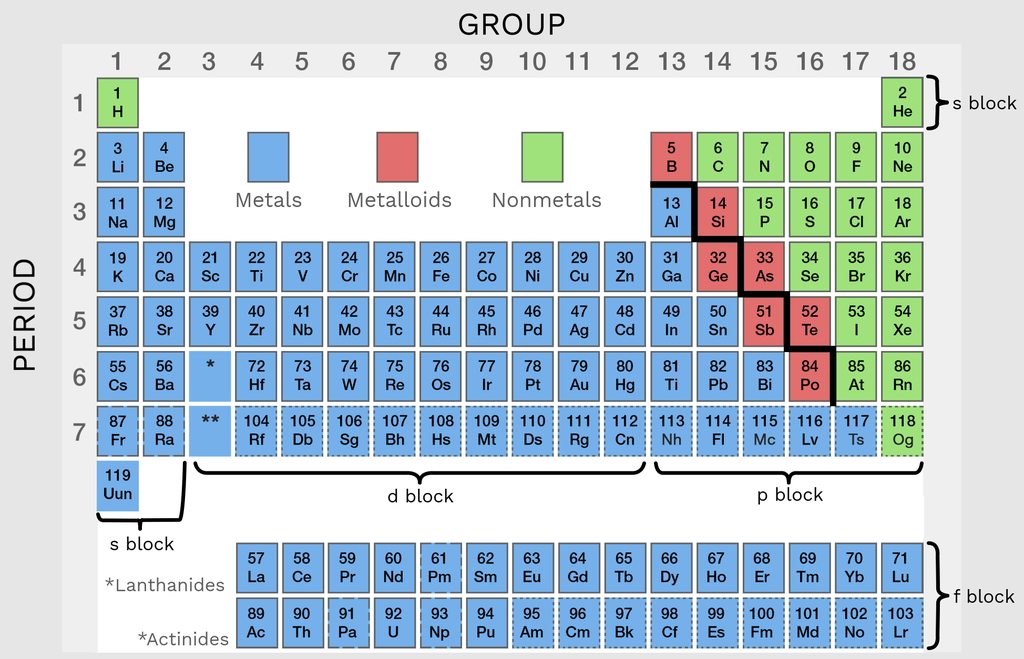The periodic table, is an organized display of all the known chemical elements, as you can see in the figure below.

Each element of the periodic table is given a period and group number. The period number defines the number of shells of electrons, while the group number correlates to the number of outer electrons (valency). The orbital these valence electrons are in, is defined by the block the element is in. We can use the period and group number, like coordinates, to locate an element on the table.

The diagonal line, starting at boron, in the p block, consists of metalloids that have the characteristics of both metals and nonmetals. If an element is found to the left of this line then it is said to be metal and so forms cations - and vice versa. Finally, if we combine the group number with the octet rule we can predict the number of bonds the element will form.

Let’s put this all into practice.

Oxygen is in group 16, so has 6 valence electrons and is right of the metal-nonmetal divide. This means oxygen will is a nonmetal and so forms an anion. The octet rule suggests this ion will have a 2 minus charge or form 2 covalent bonds.

NOTE: Transition metals, like copper and iron, can sometimes form multiple cations. We call this variable charge.Figure 1: Metals, metalloids, and nonmetals: The diagonal line cutting through the metalloids splits the table into metals, on the left, and nonmetals, on the right. Each block also organizes the elements based on their electron configuration.